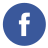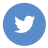### How can I set up a timed interval in javascript?

javascript

You can set up a timed interval in JavaScript using the setInterval() method. This method repeatedly calls a function or executes a code snippet at a specified time interval (in milliseconds).

Here’s an example of how to use setInterval() to call a function every 1 second:

``````// Define a function to be called at the interval
function myFunction() {
console.log("Hello, world!");
}

// Call the function every 1 second (1000 milliseconds)
setInterval(myFunction, 1000);``````

In this example, the setInterval() method is used to call the myFunction() function every 1 second. The first argument to setInterval() is the function to be called, and the second argument is the time interval in milliseconds.

You can also use an anonymous function as the first argument to setInterval() if you don’t want to define a separate function:

``````// Call an anonymous function every 1 second
setInterval(function() {
console.log("Hello, world!");
}, 1000);``````

In this example, an anonymous function is used as the first argument to setInterval(), and the function body contains the code to be executed at the interval.